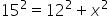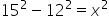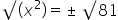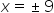Mathematics
Easy

Question

# The two sides of the right triangle are 12 units and 15 units, what will be third small side by using quadratic square roots?

## 71199Hint:

## The correct answer is: 9

### Here we have to find the third smaller side of right triangle.Firstly , we have two side which is 12 unit and 15 unit.Let the three sides be a, b and c .Then a = 12 unit , b = ? (Let x ) unit and c =15 unit.We have formula for hypotenuse,(c)2 = (a)2 + (b )2Taking square root on both the sides of the equation, we getSince the length of the side cannot be negative, x= 9 units

In this question, we have given two sides which is 12 and 15 and we have to find the third side which is smaller. So we have formula for hypotenuse which is c2 = a+ b2  but hypotenuse is largest side of the right triangle so for smaller side we can write this formula as b2 = c2 – a2 ,here we used property of equality to find this formula.

### Related Questions to study#### With Turito Foundation.#### Get an Expert Advice From Turito.